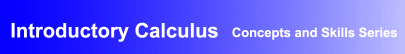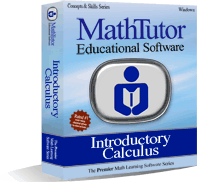Award-Winning

Calculus Interactive Learning SoftwareDESCRIPTION
INTRODUCTORY CALCULUS is the ideal

calculus learning and review academic software package.

The program covers the topics included in a one year course in differential and integral calculus.
There's no simpler way to

teach yourself calculus and to learn calculus problem solving

. Our INTRODUCTORY CALCULUS program is like a personal teacher leading you step by step through the derivative, the integral, and the many other topics that make up a comprehensive calculus tutorial program.
A

derivative calculator

is also included to provide a step by step guide to solving derivatives a student types in.
Dynamic, engaging lessons use graphics and animation to help students gain a visual understanding of the concepts of calculus and to master problem-solving.
The program is far more than a "

basic calculus for beginners

" course. It helps students gain a strong understanding of the fundamental concepts that underlie calculus, and shows students how to master calculus problem solving by applying these principles. With this strong underpinning, students are prepared for more advanced math courses, and are able to explain calculus to others.
Parents wanting a homeschool calculus interactive tutorial program will find the package to be perfectly suited to their needs.
Educators and individuals wanting interactive calculus tutorial software lessons that will help them build proficiency in problem solving will find the program to be exactly what they were looking for.
INTRODUCTORY CALCULUS has been acclaimed for its educational excellence as well as its flexibility. It can be used for self-study and to supplement classroom instruction in high school math classes. The program features an adaptive, individualized learning environment suitable for students at all levels of ability.
Designed by math educators and in accordance with math curriculum standards, the program contains tutorials, examples and calculus problem solving exercises that progress gradually from the simpler to the more challenging, allowing students to build their skills and confidence.
• Award-winning, curriculum-based calculus courseware developed by math educators
• Provides dynamic, interactive calculus lessons that are ideal for self-study, and as a supplement to classroom instruction
• Demonstrated success in boosting students' math skills and comprehension, and proficiency in problem solving
• Network versions contain student record-keeping, grading, and performance analysis features
AVAILABLE FOR WINDOWS
• Windows XP/Vista
• Windows 7/8
• Windows 10
PRICING Single User Version: \$49.95TOPICS COVERED
Prerequisites for Calculus
• Review of Powers and Exponents
• Evaluating Functions
• The Coordinate System
• The Slope of a Line
Introducing Differential Calculus
More Differential Calculus
• The Chain Rule
• Higher Order Differentiation
• Implicit Differentiation
• Max/Min Word Problems
Introducing Integral Calculus
• The Integral
• Integration of Monomials I
• Integration of Monomials II
• Integration of Sum of Monomials
• Integration of du/u
More Integral Calculus
• Area Under a Curve
• Integration by Parts
Trig., Exponential, Log Functions
• Derivative of Trig. Functions: Proofs
• Derivative of Trig. Functions: Problems
• Integration of Trig. Functions
• Differentiation and Integration of eu
• Derivative of anx